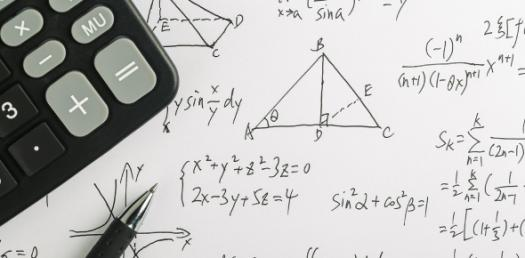# Trivia: Math Word Problems Quiz Questions!

20 Questions | Attempts: 289
ShareSettingsMath is considered by many as the toughest subject for most students, and this is far from the truth because, with enough practice, they would be able to solve any math problem they come across. So are you looking for a way to test your math knowledge? If you said yes, this quiz is exactly what you need to refresh your knowledge.

• 1.
What are the missing numbers? IN        8       24       32     19     ? OUT    16     32        40     ?    51
• A.

27 and 25

• B.

27 and 43

• C.

29 and 42

• D.

20 and 67

• 2.
What addition property is show by the following number sentence? (3+1) + 6 = 3 + (1+6)
• A.

Identity Property

• B.

Distributive Property

• C.

Associative Property

• D.

Commutative Property

• 3.
236-119 = n What does n equal?
• A.

N = 117

• B.

N = 355

• C.

N = 467

• D.

N = 118

• 4.
• A.

• B.

Any number that is subtracted or added.

• C.

• D.

The answer to a subtraction problem.

• 5.
What addition property is shown by the following number sentence? 4 + 6 = 6 + 4
• A.

Identity Property

• B.

Commutative Property

• C.

Distributive Property

• D.

Associative Property

• 6.
329 + 468 =
• 7.
800 - 473 =
• 8.
Complete the fact family for the number 7, 13, and 6. 7 + 6 = 13 13 - 6 = 7 6 + 7 = 13 ? ? ? ? ?
• 9.
How much is 4 hundreds, 4 tens, and 16 ones?
• 10.
Write a number sentence to solve the following problem, use "n" as your variable for what you are trying to find.  (do not answer the question, "n" will be in place of the answer). Pam and Bob went to the store to buy groceries for their mother.  They have \$40 to spend and the bill came out to \$27.43.  How much change will they get?
• 11.
Estimate to solve.  Write the estimated sum and overestimate or underestimate your answer. 568 - 335
• 12.
Tell whether the following problem would be an overestimate or an underestimate. 498 + 334
• 13.
Round to the nearest hundred to estimate the difference.  Write the estimated difference. 1,245 - 456
• 14.
What is the sum of 456 and 523?
• 15.
What is the sum of 947 and 328?
• 16.
What is 500 - 328?
• 17.
Which number would make this number sentence TRUE? 26 + y < 34
• A.

Y = 4

• B.

Y = 10

• C.

Y = 8

• D.

Y = 13

• 18.
What symbol would fit in this number sentence? 20 ____  13 + 9
• A.

• B.

>

• C.

+

• D.

=

• 19.
What is 234 - 136?
• 20.
What is \$23.87 + \$19.32?

## Related TopicsBack to top
×

Wait!
Here's an interesting quiz for you.